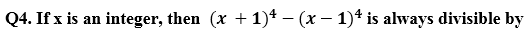Dear Students!!! There is most general as well as a scoring section in all the competitive entrance examinations in the teaching field i.e "Mathematics".Because in this section only one thing is work i.e your accuracy and that could be nourished with the daily practice. So, for this, we are providing you the daily quiz for all teaching exams i.e CTET Exam 2019, DSSSB ,KVS,STET Exam.

Preparing For CTET 2019? Enroll Now For CTET Prime :
Use This Code TEACH5(a) 90.903
(b) 16.2903
(c) 15.2903
(d) 91.903(a) 6
(b) 8
(c) 9
(d) 12

Q5. The hundred digit of a three digit number is 7 more than the unit digit. The digits of the number are reversed and the resulting number is subtracted from the original three digit number. The unit digit of the final number, so obtained is

(a) 0
(b) 1
(c) 2
(d) 3

Q6. If a, b and c are different integers such that a < b < c < 0, then which of the following statements is true ?

(a) a + c < b
(b) ab < c
(c) a + b > c
(d) ac > abQ8. Which one of the following statements is correct ?

(a) sum of two prime numbers is always a prime number
(b) 1 is the smallest prime number
(c) a composite number can be odd
(d) there is no even prime number

Q9. The values of y for which the 4-digit number 51y3 is divisible by 9 is

(a) 2 or 3
(b) 0 or 9
(c) 0 or 3
(d) 3 or 9

Q10. The product of integers between -7 and – 3 is

(a) 120
(b) –360
(c) –120

You may also like to read :